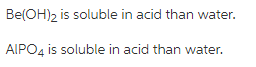# Which salts will be more soluble in an acidic solution than in pure water

Which salts will be more soluble in an acidic solution than in pure water? Explain why each salt is or is not more soluble in an acidic solution than in pure water.

CuCl
CsClO4
CaSO4
Be(OH)2
AlPO4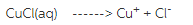Acid gives H+ ions . H+ ions combine to gives strong acid HCl. So the equilibrium shift to left side . It is insoluble in acid solution.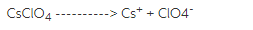Acid gives H+ ions . H+ ions combine to gives strong acid HClO4. So the equilibrium shift to left side . It is insoluble in acid solution.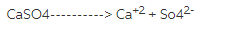Acid gives H+ ions . H+ ions combine to gives strong acid H2So4. So the equilibrium shift to left side . It is insoluble in acid solution.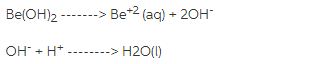Acid gives H+ ions . H+ ions combine to gives unionisable H2O. So the equilibrium shift to left side . It is insoluble in acid solution.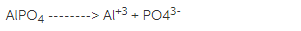Acid gives H+ ions . H+ ions combine to gives weak acid H3PO4. So the equilibrium shift to right side . It is soluble in acid solution.